Note: The other languages of the website are Google-translated. Back to English

## Sumif with multiple criteria based on OR and AND logic

When working on Excel worksheets, you may need to sum values based on multiple criteria. Sometimes, the multiple criteria are from the same column (OR logic), but sometimes from different columns (AND logic). In this case, how could you deal with this task in Excel?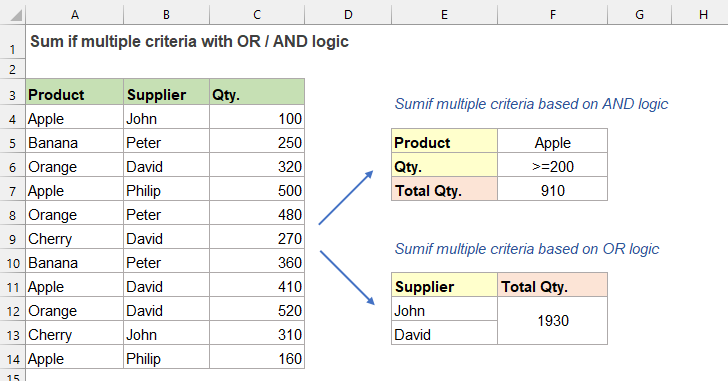#### Sumif with multiple criteria based on OR logic

Supposing, you have a product list which contains the Supplier and Qty as below screenshot shown, now, you want to get all total quantities that are supplied by the supplier John and David. Here, I will introduce two simple formulas for you.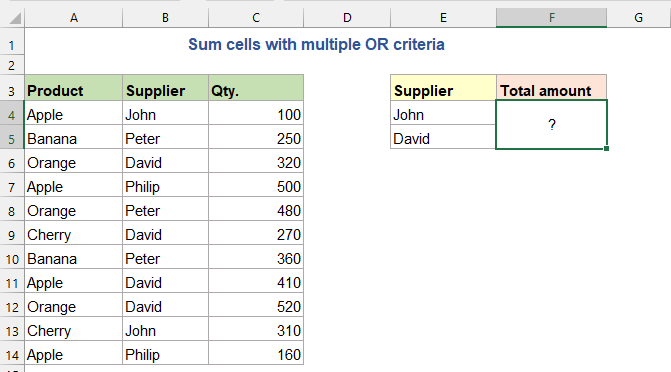##### By using SUMIF + SUMIF +…formula

If you want to sum numbers that meet either of the criteria (OR logic) from multiple criteria, you can add up several SUMIF functions in a single formula, the generic syntax is:

=SUMIF(criteria_range, criteria1, sum_range)+SUMIF(criteria_range, criteria2, sum_range)+…
• criteria_range: The range of cells you want to match the criteria;
• criteria1: The first criteria that is used to determine which cells should be summed;
• criteria2: The second criteria that is used to determine which cells should be summed;
• sum_range: The range of cells you want to sum from.

Now, please copy or enter any one of the below formulas into a blank cell, and press Enter key to get the result:

=SUMIF(B4:B14,"John",C4:C14) + SUMIF(B4:B14,"David",C4:C14)           (Type the criteria manually)
=SUMIF(B4:B14,E4,C4:C14) + SUMIF(B4:B14,E5,C4:C14)
(Use a cell reference)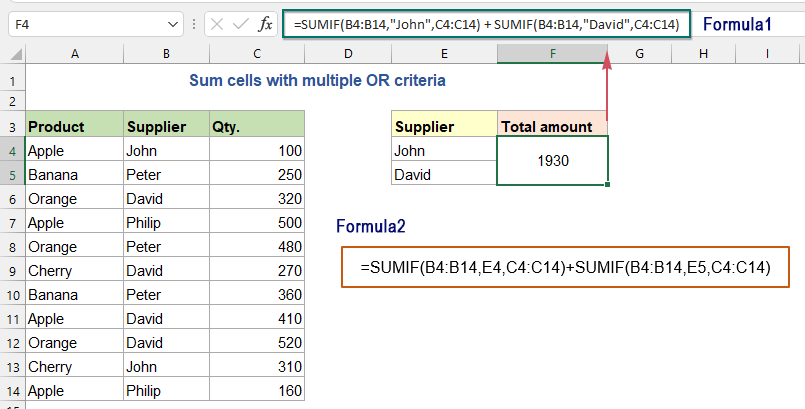##### Explanation of the formula:

=SUMIF(B4:B14,"John",C4:C14) + SUMIF(B4:B14,"David",C4:C14)

• The first SUMIF(B4:B14,"John",C4:C14) finds the rows of John and sum the total quantities;
• The second SUMIF(B4:B14,"David",C4:C14) finds the rows of David and sum the total quantities;
• Then add up these two SUMIF formulas to get all total quantities supplied by both John and David.

##### By using SUM and SUMIF functions

The above formula is very easy to use if there are only a couple of criteria, but if you want to sum values with multiple OR conditions, the above formula could be redundant. In this case, a better formula which is created based on SUM and SUMIF functions may do you a favor. The generic syntaxes are:

Generic formula with hardcoded text:

=SUM(SUMIF(criteria_range, {criteria1,criteria2,...}, sum_range))
• criteria_range: The range of cells you want to match the criteria;
• criteria1: The first criteria that is used to determine which cells should be summed;
• criteria2: The second criteria that is used to determine which cells should be summed;
• sum_range: The range of cells you want to sum from.

Generic formula with cell references:

{=SUM(SUMIF(criteria_range, criteria_cells, sum_range))}
Array formula, should press Ctrl + Shift + Enter keys together.
• criteria_range: The range of cells you want to match the criteria;
• criteria_cells: The cells that contain the criteria you want to use;
• sum_range: The range of cells you want to sum from.

Please, enter or copy any one of the below formulas you need into a blank cell, and then get the result:

=SUM(SUMIF(B4:B14, {"John","David"}, C4:C14))           (Type the criteria manually)
=SUM(SUMIF(B4:B14, E4:E5, C4:C14))
(Use cell references, array formula, should press Ctrl + Shift + Enter keys)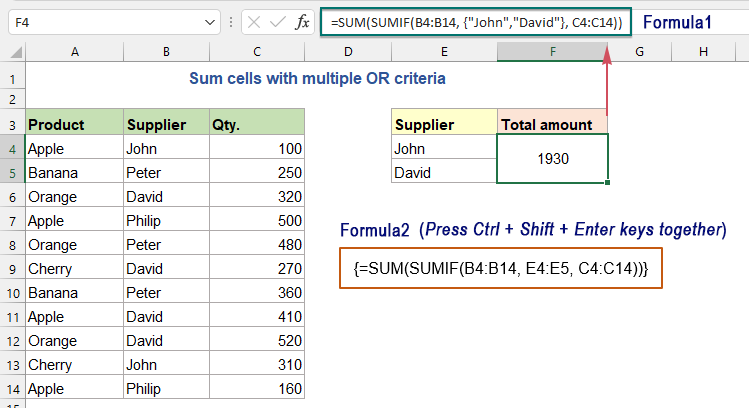##### Explanation of the formula:

=SUM(SUMIF(B4:B14, {"John","David"}, C4:C14))

> SUMIF(B4:B14, {"John","David"}, C4:C14):

• {"John","David"}: An array constant which is a set of multiple criteria provided in curly braces.
• SUMIF(B4:B14,"David",C4:C14) The array constant using OR logic forces SUMIF function to sum numbers in C4:C14 based on either of the multiple criteria ("John" and "David"), and it will return two separate results: {410,1520}.

> SUM(SUMIF(B4:B14, {"John","David"}, C4:C14))=SUM({410,1520}): Finally, this SUM function adds up these array results to return the result: 1930.

#### Sumif with multiple criteria based on AND logic by using the SUMIFS function

If you want to sum values with multiple criteria in different columns, you can use the SUMIF function to solve this task quickly. The generic syntax is:

=SUMIFS(sum_range, criteria_range1, criteria1, [criteria_range2, criteria2], ...)
• sum_range: The range of cells you want to sum from;
• criteria_range1: The range where criteira1 is applied;
• criteria1: The first criteria that is checked against the criteria_range1 and determines which cells to add; (the type of criteria can be: number, logical expression, cell reference, text, date or other Excel function.)
• criteria_range2, criteria2…: Other additional ranges and their associated criteria. (you can set up 127 pairs of criteria_range and criteria in a SUMIFS formula.)

Let’s say, I have a table with Product, Supplier, Qty and Price columns as below screenshot shown. Now, I want to find out the sum of total price of the product Apple and the quantity that is greater than or equal to 200.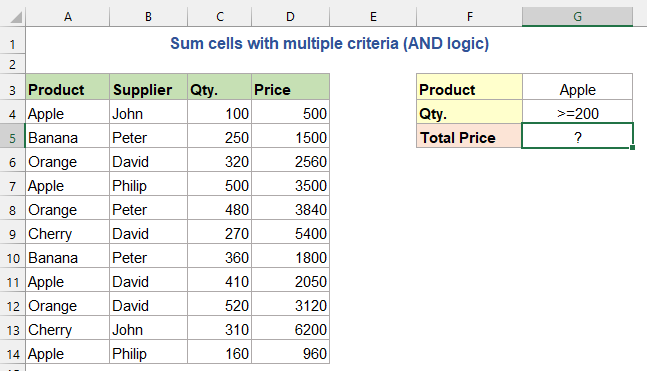Please apply any one of the below formulas into a blank cell, and press Enter key to return the result:

=SUMIFS(D4:D14,A4:A14,"Apple",C4:C14,">=200")           (Type the criteria manually)
=SUMIFS(C4:C14,A4:A14,G3,C4:C14,G4)
(Use cell references)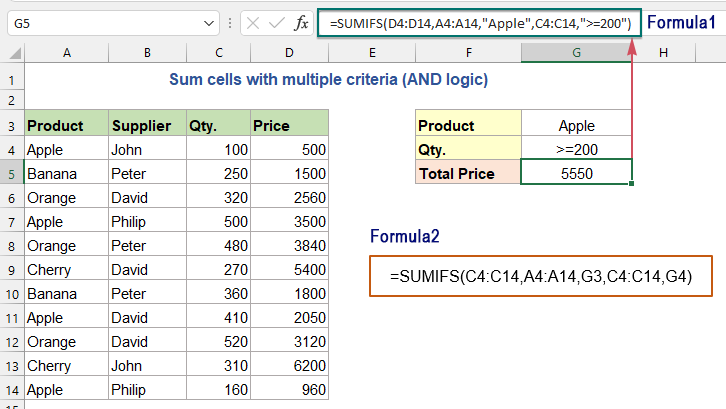##### Explanation of the formula:

=SUMIFS(D4:D14,A4:A14,"Apple",C4:C14,">=200")

• The range A4:A14 checks for the product “Apple”, and the range C4:C14 extracts all quantities greater than or equal to 200, then the SUMIFS function sums only the matched cells from the range D4:D14.

#### Relative function used:

• SUMIF:
• The SUMIF function can help to sum cells based on one criterion.
• SUMIFS:
• The SUMIFS function in Excel can help to sum cell values based on multiple criteria.

#### More articles:

• Sum If Cells Contain Specific Text In Another Column
• This tutorial will show you how to sum values if cells contain specific or partial text in another column. Take the below data range as an example, to get the total amounts of the products containing the text “T-Shirt”, both the SUMIF function and SUMPRODUCT function can deal with this task in Excel.
• Sum Smallest Or Bottom N Values In Excel
• In Excel, it is easy for us to sum a range of cells by using the SUM function. Sometimes, you may need to sum the smallest or bottom 3, 5 or n numbers in a data range as below screenshot shown. In this case, the SUMPRODUCT together with the SMALL function can help you to solve this problem in Excel.

### The Best Office Productivity Tools

#### Kutools for Excel - Helps You To Stand Out From Crowd

Would you like to complete your daily work quickly and perfectly? Kutools for Excel brings 300 powerful advanced features (Combine workbooks, sum by color, split cell contents, convert date, and so on...) and save 80% time for you.

• Designed for 1500 work scenarios, helps you solve 80% Excel problems.
• Reduce thousands of keyboard and mouse clicks every day, relieve your tired eyes and hands.
• Become an Excel expert in 3 minutes. No longer need to remember any painful formulas and VBA codes.
• 30-day unlimited free trial. 60-day money back guarantee. Free upgrade and support for 2 years.#### Office Tab - Enable Tabbed Reading and Editing in Microsoft Office (include Excel)

• One second to switch between dozens of open documents!
• Reduce hundreds of mouse clicks for you every day, say goodbye to mouse hand.
• Increases your productivity by 50% when viewing and editing multiple documents.
• Brings Efficient Tabs to Office (include Excel), Just Like Chrome, Firefox, And New Internet Explorer.